# 8th Grade - Special Right Triangles

## Introduction

• Aside from the Pythagorean Theorem, there are helpful theorems we can use to solve for the measure of side lengths or angles of a right triangle.
• Two special triangles are important in geometry - $30°-60°-90°$ Triangle, and $45°-45°-90°$ Triangle.
• $45°-45°-90°$ Triangle is also called the Isosceles Right Triangle.

## Theorems on Special Right Triangles

• $\mathbf{30}\mathbf{°}\mathbf{-}\mathbf{60}\mathbf{°}\mathbf{-}\mathbf{90}\mathbf{°}$ Triangle Theorem - In $30°-60°-90°$ Triangle, the side opposite the $30°$ angle is half as long as the hypotenuse, and the side opposite the $60°$ angle is $\sqrt{3}$ times as long as the side opposite the $30°$ angle.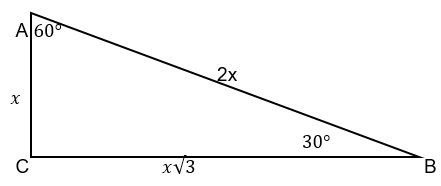• The converse of the $\mathbf{30}\mathbf{°}\mathbf{-}\mathbf{60}\mathbf{°}\mathbf{-}\mathbf{90}\mathbf{°}$ Triangle - If one leg is half as long as the hypotenuse in a right triangle, then the opposite angle has a measure of 30 degrees.
• Isosceles Right Triangle Theorem - In an isosceles right triangle, the hypotenuse is $\sqrt{2}$ times as long as either of the legs. To illustrate, we have $AC=BC$ with hypotenuse $AB=x\sqrt{2}$.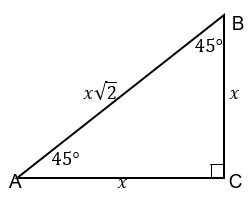## Solved Examples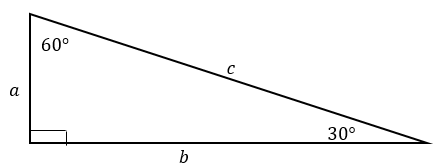Example 1. Refer to the figure above. If $c=30$, what is the length of $a$ and $b$?

Solution:

Since the length of the hypotenuse is $30$ units, the length of $a$ is $15$ units.

If $a=15$ units, then $b=15\sqrt{3}$ units.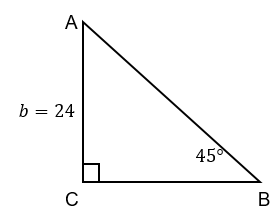Example 2. Refer to the figure above. If the length of leg $b$ is 24 units, what are the lengths of the hypotenuse and the other leg?

Solution:

$△ACB$ is an isosceles right triangle.

If one leg measures 24 units, the other leg is 24 units.

The length of the hypotenuse is $24\sqrt{2}$ units.

## Other Theorems on Right Triangles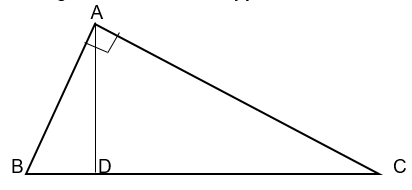• In a right triangle, the altitude to the hypotenuse divides the triangle into two similar triangles to the original triangle.
• In any right triangle, the altitude to the hypotenuse is the geometric mean between the segments into which it divides the hypotenuse. To illustrate, we have the equation $\frac{BD}{AD}=\frac{AD}{CD}$.
• Each leg is a geometric mean between the hypotenuse and the hypotenuse segment adjacent to the leg. To illustrate, we have the following equations: $\frac{BC}{AB}=\frac{AB}{BD}$ and $\frac{BC}{AC}=\frac{AC}{CD}$

## Cheat Sheet

• In the 30-60-90 Triangle, if the longer leg $a$ has a measure $x$ and the hypotenuse is denoted by $c$, then $c=2x$ and $b=x\sqrt{3}$ units.
• In the 45-45-90 Triangle, if one leg measures $x$, then the other leg measures $x$ and the hypotenuse measures $x\sqrt{2}$ units.
• The side opposite the 30-degree angle is the shorter leg.
• The side opposite the 60-degree angle is the longer leg.
• The angle opposite the shorter leg measures $30°$.
• The angle opposite the longer leg measures $60°$.

## Blunder Areas

• The sides of a right triangle are not closed using the symbols or $c$. Any letter symbol can be used as long as the concepts of the theorems are appropriately utilized.
• It is typical that uppercase letters are used to denote angles while lowercase letters are used to denote side lengths of a right triangle.
• 30-60-90 Triangle Theorem is used to derive the formula of the Area of an Equilateral Triangle, $A=\frac{{s}^{2}\sqrt{3}}{4}$.
• The Isosceles Triangle Theorem and Median Theorem of a right triangle can be used in proving the 30-60-90 Triangle Theorem.
• Pythagorean Theorem can be used in proving the 45-45-90 Triangle Theorem.